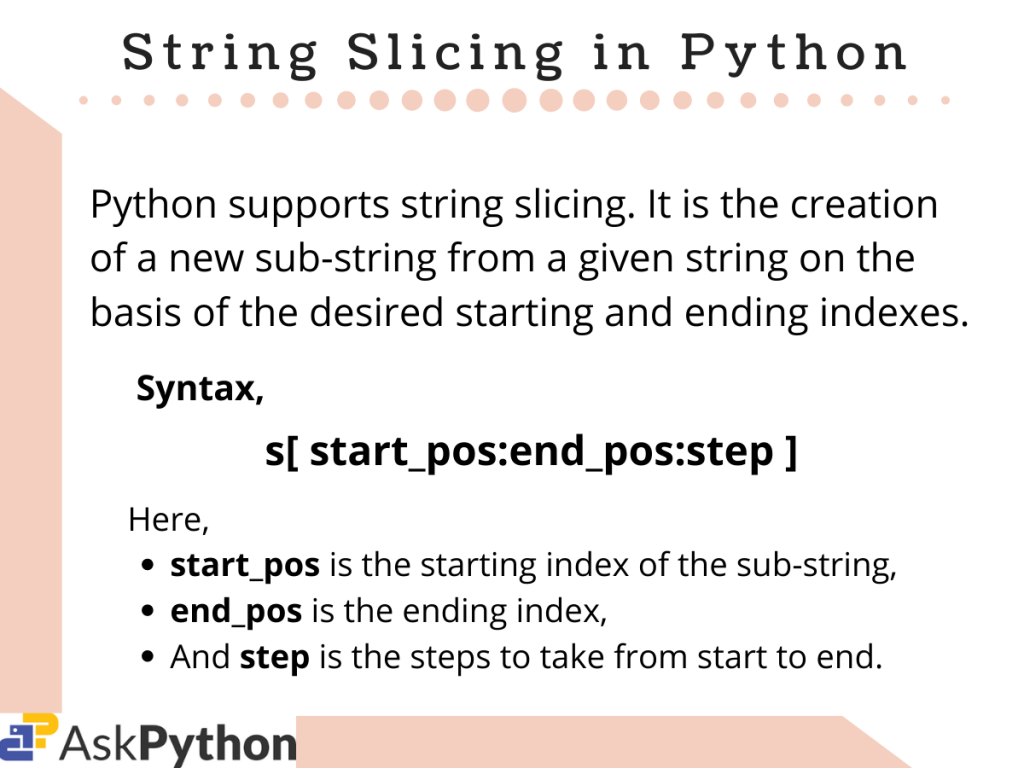# How to Slice Strings in Python?## Introduction

In this tutorial, we are going to learn how we can slice strings in Python.

Python supports the slicing of strings. It is the creation of a new sub-string from the given string on the basis of the user-defined starting and ending indices.

## Ways to Slice Strings in Python

If you want to slice strings in Python, it’s going to be as simple as this one line below.

```res_s = s[ start_pos:end_pos:step ]
```

Here,

• res_s stores the returned sub-string,
• s is the given string,
• start_pos is the starting index from which we need to slice the string s,
• end_pos is the ending index, before which the slicing operation would end,
• step is the steps the slicing process would take from start_pos to end_pos.

Note: All of the above three parameters are optional. By default, `start_pos` is set to 0, `end_pos` is considered equal to the length of the string, and `step` is set to 1.

Now let us take some examples to understand how to slice strings in Python in a better way.

## Slice Strings in Python – Examples

Slicing Python strings can be done in different ways.

Usually, we access string elements(characters) using simple indexing that starts from 0 up to n-1(n is the length of the string). Hence for accessing the 1st element of a string `string1`, we can simply use the below code.

```s1 = String1
```

Again, there is another way to access these characters, that is, using negative indexing. Negative indexing starts from -1 to -n(n is the length for the given string). Note, negative indexing is done from the other end of the string. Hence, to access the first character this time we need to follow the below-given code.

```s1 = String﻿1[-n]
```

Now let us look at some ways following which we can slice a string using the above concept.

### 1. Slice Strings in Python with Start and End

We can easily slice a given string by mentioning the starting and ending indices for the desired sub-string we are looking for. Look at the example given below, it explains string slicing using starting and ending indices for both usual and negative indexing method.

```#string slicing with two parameters
s = "Hello World!"

res1 = s[2:8]
res2 = s[-4:-1] #using negative indexing

print("Result1 = ",res1)
print("Result2 = ",res2)
```

Output:

```Result1 =  llo Wo
Result2 =  rld
```

Here,

• We initialize a string, `s` as “Hello World!”,
• At first, we slice the given string with starting index 2 and ending index as 8. This means that the resultant sub-string would contain the characters from s to s[8-1],
• Similarly, for the next one, the resultant sub-string should contain characters from s[-4] to s[(-1)-1].

Hence, our output is justified.

### 2. Slice Strings Using Only the Start or End

As mentioned earlier, all of the three parameters for string slicing are optional. Hence, we can easily accomplish our tasks using one parameter. Look at the code below to get a clear understanding.

```#string slicing with one parameter
s1= "Charlie"
s2="Jordan"

res1 = s1[2:] #default value of ending position is set to the length of string
res2 = s2[:4] #default value of starting position is set to 0

print("Result1 = ",res1)
print("Result2 = ",res2)
```

Output:

```Result1 =  arlie
Result2 =  Jord
```

Here,

• We firstly initialize two strings, s1 and s2,
• For slicing both of them we just mention the start_pos for s1, and end_pos for s2 only,
• Hence for res1, it contains a sub-string of s1 from index 2(as mentioned) up to the last(by default it is set to n-1). Whereas for res2, the range of the indices lies from 0 upto 4(mentioned).

### 3. Slice Strings in Python with Step Parameter

The `step` value decides the jump the slicing operation would take from one index to the other. Look at the example below carefully.

```#string slicing with step parameter
s= "Python"
s1="Kotlin"

res = s[0:5:2]
res1 = s1[-1:-4:-2] #using negative parameters

print("Resultant sliced string = ",res)
print("Resultant sliced string(negative parameters) = ",res1)
```

Output:

```Resultant sliced string =  Pto
Resultant sliced string(negative parameters) =  nl
```

In the code above,

• We initialize two strings s and s1, and try to slice them for the given starting and ending indices as we did for our first example,
• But this time we have mentioned a step value which was set to 1 by default for the previous examples,
• For res, having a step size 2 means, that while the traversal to get the substring from index 0 to 4, each time the index would be increased by a value of 2. That is the first character is s (‘P’), the next characters in the sub-string would be s[0+2], and s[2+2], until the index is just less than 5.
• For the next one i.e. res1, the step mentioned is (-2). Hence, similar to the previous case, the characters in the substring would be s1[-1] , then s1[(-1)+(-2)] or s1[-3] until the index is just less than (-4).

### 4. Reversing a String using Slicing in Python

With the use of negative index string slicing in Python, we can also reverse the string and store it in another variable. For doing so we just need to mention a `step` size of (-1).

Let us see how that works in the example given below.

```#reversing string using string slicing
rev_s = s[::-1] #reverse string stored into rev_s

print(rev_s)
```

Output:

```nohtyPksA
```

As we can see, the string s is reversed and stored into `rev_s`. Note: For this case too, the original string remains intact and untouched.

## Conclusion

So in this tutorial, we learned about the string slicing methodology and its different forms. Hope, the readers had a clear understanding of the topic.

For any further questions related to this topic, feel free to use the comments below.